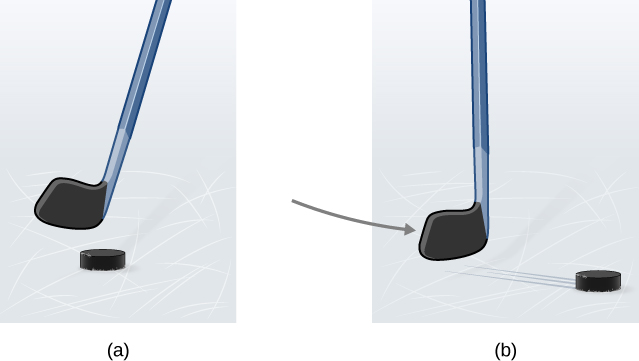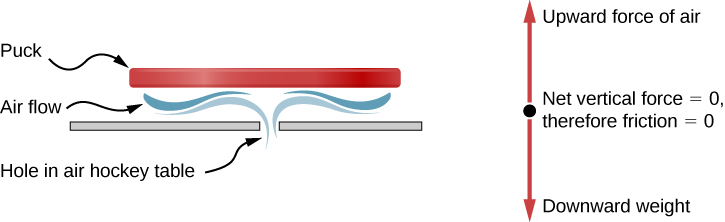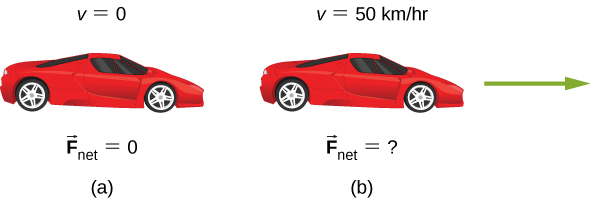## 5.2 Newton’s First Law

### Learning Objectives

By the end of the section, you will be able to:

• Describe Newton’s first law of motion
• Recognize friction as an external force
• Define inertia
• Identify inertial reference frames
• Calculate equilibrium for a system

Experience suggests that an object at rest remains at rest if left alone and that an object in motion tends to slow down and stop unless some effort is made to keep it moving. However, Newton’s first law gives a deeper explanation of this observation.

#### Newton’s First Law of Motion

A body at rest remains at rest or, if in motion, remains in motion at constant velocity unless acted on by a net external force.

Note the repeated use of the verb “remains.” We can think of this law as preserving the status quo of motion. Also note the expression “constant velocity;” this means that the object maintains a path along a straight line, since neither the magnitude nor the direction of the velocity vector changes. We can use (Figure) to consider the two parts of Newton’s first law.Figure 5.7 (a) A hockey puck is shown at rest; it remains at rest until an outside force such as a hockey stick changes its state of rest; (b) a hockey puck is shown in motion; it continues in motion in a straight line until an outside force causes it to change its state of motion. Although it is slick, an ice surface provides some friction that slows the puck.

Rather than contradicting our experience, Newton’s first law says that there must be a cause for any change in velocity (a change in either magnitude or direction) to occur. This cause is a net external force, which we defined earlier in the chapter. An object sliding across a table or floor slows down due to the net force of friction acting on the object. If friction disappears, will the object still slow down?

The idea of cause and effect is crucial in accurately describing what happens in various situations. For example, consider what happens to an object sliding along a rough horizontal surface. The object quickly grinds to a halt. If we spray the surface with talcum powder to make the surface smoother, the object slides farther. If we make the surface even smoother by rubbing lubricating oil on it, the object slides farther yet. Extrapolating to a frictionless surface and ignoring air resistance, we can imagine the object sliding in a straight line indefinitely. Friction is thus the cause of slowing (consistent with Newton’s first law). The object would not slow down if friction were eliminated.

Consider an air hockey table ((Figure)). When the air is turned off, the puck slides only a short distance before friction slows it to a stop. However, when the air is turned on, it creates a nearly frictionless surface, and the puck glides long distances without slowing down. Additionally, if we know enough about the friction, we can accurately predict how quickly the object slows down.Figure 5.8 An air hockey table is useful in illustrating Newton’s laws. When the air is off, friction quickly slows the puck; but when the air is on, it minimizes contact between the puck and the hockey table, and the puck glides far down the table.

Newton’s first law is general and can be applied to anything from an object sliding on a table to a satellite in orbit to blood pumped from the heart. Experiments have verified that any change in velocity (speed or direction) must be caused by an external force. The idea of generally applicable or universal laws is important—it is a basic feature of all laws of physics. Identifying these laws is like recognizing patterns in nature from which further patterns can be discovered. The genius of Galileo, who first developed the idea for the first law of motion, and Newton, who clarified it, was to ask the fundamental question: “What is the cause?” Thinking in terms of cause and effect is fundamentally different from the typical ancient Greek approach, when questions such as “Why does a tiger have stripes?” would have been answered in Aristotelian fashion, such as “That is the nature of the beast.” The ability to think in terms of cause and effect is the ability to make a connection between an observed behavior and the surrounding world.

### Gravitation and Inertia

Regardless of the scale of an object, whether a molecule or a subatomic particle, two properties remain valid and thus of interest to physics: gravitation and inertia. Both are connected to mass. Roughly speaking, mass is a measure of the amount of matter in something. Gravitation is the attraction of one mass to another, such as the attraction between yourself and Earth that holds your feet to the floor. The magnitude of this attraction is your weight, and it is a force.

Mass is also related to inertia, the ability of an object to resist changes in its motion—in other words, to resist acceleration. Newton’s first law is often called the law of inertia. As we know from experience, some objects have more inertia than others. It is more difficult to change the motion of a large boulder than that of a basketball, for example, because the boulder has more mass than the basketball. In other words, the inertia of an object is measured by its mass. The relationship between mass and weight is explored later in this chapter.

### Inertial Reference Frames

Earlier, we stated Newton’s first law as “A body at rest remains at rest or, if in motion, remains in motion at constant velocity unless acted on by a net external force.” It can also be stated as “Every body remains in its state of uniform motion in a straight line unless it is compelled to change that state by forces acting on it.” To Newton, “uniform motion in a straight line” meant constant velocity, which includes the case of zero velocity, or rest. Therefore, the first law says that the velocity of an object remains constant if the net force on it is zero.

Newton’s first law is usually considered to be a statement about reference frames. It provides a method for identifying a special type of reference frame: the inertial reference frame. In principle, we can make the net force on a body zero. If its velocity relative to a given frame is constant, then that frame is said to be inertial. So by definition, an inertial reference frame is a reference frame in which Newton’s first law is valid. Newton’s first law applies to objects with constant velocity. From this fact, we can infer the following statement.

#### Inertial Reference Frame

A reference frame moving at constant velocity relative to an inertial frame is also inertial. A reference frame accelerating relative to an inertial frame is not inertial.

Are inertial frames common in nature? It turns out that well within experimental error, a reference frame at rest relative to the most distant, or “fixed,” stars is inertial. All frames moving uniformly with respect to this fixed-star frame are also inertial. For example, a nonrotating reference frame attached to the Sun is, for all practical purposes, inertial, because its velocity relative to the fixed stars does not vary by more than one part in ${10}^{10}.$ Earth accelerates relative to the fixed stars because it rotates on its axis and revolves around the Sun; hence, a reference frame attached to its surface is not inertial. For most problems, however, such a frame serves as a sufficiently accurate approximation to an inertial frame, because the acceleration of a point on Earth’s surface relative to the fixed stars is rather small ($<3.4\,×\,{10}^{-2}\,{\text{m/s}}^{2}$). Thus, unless indicated otherwise, we consider reference frames fixed on Earth to be inertial.

Finally, no particular inertial frame is more special than any other. As far as the laws of nature are concerned, all inertial frames are equivalent. In analyzing a problem, we choose one inertial frame over another simply on the basis of convenience.

### Newton’s First Law and Equilibrium

Newton’s first law tells us about the equilibrium of a system, which is the state in which the forces on the system are balanced. Returning to Forces and the ice skaters in (Figure), we know that the forces ${\overset{\to }{F}}_{1}$ and ${\overset{\to }{F}}_{2}$ combine to form a resultant force, or the net external force: ${\overset{\to }{F}}_{\text{R}}={\overset{\to }{F}}_{\text{net}}={\overset{\to }{F}}_{1}+{\overset{\to }{F}}_{2}.$ To create equilibrium, we require a balancing force that will produce a net force of zero. This force must be equal in magnitude but opposite in direction to ${\overset{\to }{F}}_{\text{R}},$ which means the vector must be $\text{−}{\overset{\to }{F}}_{\text{R}}.$ Referring to the ice skaters, for which we found ${\overset{\to }{F}}_{\text{R}}$ to be $30.0\hat{i}+40.0\hat{j}\,\text{N}$, we can determine the balancing force by simply finding $\text{−}{\overset{\to }{F}}_{\text{R}}=-30.0\hat{i}-40.0\hat{j}\,\text{N}.$ See the free-body diagram in (Figure)(b).

We can give Newton’s first law in vector form:

$\overset{\to }{v}=\text{constant when}\,{\overset{\to }{F}}_{\text{net}}=\overset{\to }{0}\,\text{N}.$

This equation says that a net force of zero implies that the velocity $\overset{\to }{v}$ of the object is constant. (The word “constant” can indicate zero velocity.)

Newton’s first law is deceptively simple. If a car is at rest, the only forces acting on the car are weight and the contact force of the pavement pushing up on the car ((Figure)). It is easy to understand that a nonzero net force is required to change the state of motion of the car. However, if the car is in motion with constant velocity, a common misconception is that the engine force propelling the car forward is larger in magnitude than the friction force that opposes forward motion. In fact, the two forces have identical magnitude.Figure 5.9 A car is shown (a) parked and (b) moving at constant velocity. How do Newton’s laws apply to the parked car? What does the knowledge that the car is moving at constant velocity tell us about the net horizontal force on the car?

### Example

#### When Does Newton’s First Law Apply to Your Car?

Newton’s laws can be applied to all physical processes involving force and motion, including something as mundane as driving a car.

(a) Your car is parked outside your house. Does Newton’s first law apply in this situation? Why or why not?

(b) Your car moves at constant velocity down the street. Does Newton’s first law apply in this situation? Why or why not?

#### Strategy

In (a), we are considering the first part of Newton’s first law, dealing with a body at rest; in (b), we look at the second part of Newton’s first law for a body in motion.

#### Solution

1. When your car is parked, all forces on the car must be balanced; the vector sum is 0 N. Thus, the net force is zero, and Newton’s first law applies. The acceleration of the car is zero, and in this case, the velocity is also zero.
2. When your car is moving at constant velocity down the street, the net force must also be zero according to Newton’s first law. The car’s engine produces a forward force; friction, a force between the road and the tires of the car that opposes forward motion, has exactly the same magnitude as the engine force, producing the net force of zero. The body continues in its state of constant velocity until the net force becomes nonzero. Realize that a net force of zero means that an object is either at rest or moving with constant velocity, that is, it is not accelerating. What do you suppose happens when the car accelerates? We explore this idea in the next section.

#### Significance

As this example shows, there are two kinds of equilibrium. In (a), the car is at rest; we say it is in static equilibrium. In (b), the forces on the car are balanced, but the car is moving; we say that it is in dynamic equilibrium. (We examine this idea in more detail in Static Equilibrium and Elasticity.) Again, it is possible for two (or more) forces to act on an object yet for the object to move. In addition, a net force of zero cannot produce acceleration.

A skydiver opens his parachute, and shortly thereafter, he is moving at constant velocity. (a) What forces are acting on him? (b) Which force is bigger?

Engage this simulation to predict, qualitatively, how an external force will affect the speed and direction of an object’s motion. Explain the effects with the help of a free-body diagram. Use free-body diagrams to draw position, velocity, acceleration, and force graphs, and vice versa. Explain how the graphs relate to one another. Given a scenario or a graph, sketch all four graphs.

### Summary

• According to Newton’s first law, there must be a cause for any change in velocity (a change in either magnitude or direction) to occur. This law is also known as the law of inertia.
• Friction is an external force that causes an object to slow down.
• Inertia is the tendency of an object to remain at rest or remain in motion. Inertia is related to an object’s mass.
• If an object’s velocity relative to a given frame is constant, then the frame is inertial. This means that for an inertial reference frame, Newton’s first law is valid.
• Equilibrium is achieved when the forces on a system are balanced.
• A net force of zero means that an object is either at rest or moving with constant velocity; that is, it is not accelerating.

### Conceptual Questions

Taking a frame attached to Earth as inertial, which of the following objects cannot have inertial frames attached to them, and which are inertial reference frames?

(a) A car moving at constant velocity

(b) A car that is accelerating

(c) An elevator in free fall

(d) A space capsule orbiting Earth

(e) An elevator descending uniformly

A woman was transporting an open box of cupcakes to a school party. The car in front of her stopped suddenly; she applied her brakes immediately. She was wearing her seat belt and suffered no physical harm (just a great deal of embarrassment), but the cupcakes flew into the dashboard and became “smushcakes.” Explain what happened.

### Problems

Two forces of ${\overset{\to }{F}}_{1}=\frac{75.0}{\sqrt{2}}(\hat{i}-\hat{j})\,\text{N}$ and ${\overset{\to }{F}}_{2}=\frac{150.0}{\sqrt{2}}(\hat{i}-\hat{j})\,\text{N}$ act on an object. Find the third force ${\overset{\to }{F}}_{3}$ that is needed to balance the first two forces.

While sliding a couch across a floor, Andrea and Jennifer exert forces ${\overset{\to }{F}}_{\text{A}}$ and ${\overset{\to }{F}}_{\text{J}}$ on the couch. Andrea’s force is due north with a magnitude of 130.0 N and Jennifer’s force is $32\text{°}$ east of north with a magnitude of 180.0 N. (a) Find the net force in component form. (b) Find the magnitude and direction of the net force. (c) If Andrea and Jennifer’s housemates, David and Stephanie, disagree with the move and want to prevent its relocation, with what combined force ${\overset{\to }{F}}_{\text{DS}}$ should they push so that the couch does not move?

### Glossary

inertia
ability of an object to resist changes in its motion
inertial reference frame
reference frame moving at constant velocity relative to an inertial frame is also inertial; a reference frame accelerating relative to an inertial frame is not inertial
law of inertia
see Newton’s first law of motion
Newton’s first law of motion
body at rest remains at rest or, if in motion, remains in motion at constant velocity unless acted on by a net external force; also known as the law of inertia